## FIRST Q：系统及显卡版本问题！!!!

win10 显卡N960M,Python3.6.9（aconda环境）

tensorflow_gpu==1.5.0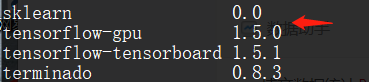TensorFlow-gpu版本与CUDA以及CUDNN版本要保持一致
CUDA-win10-9.0  CUDNN-7.0.4-FourCuda9.0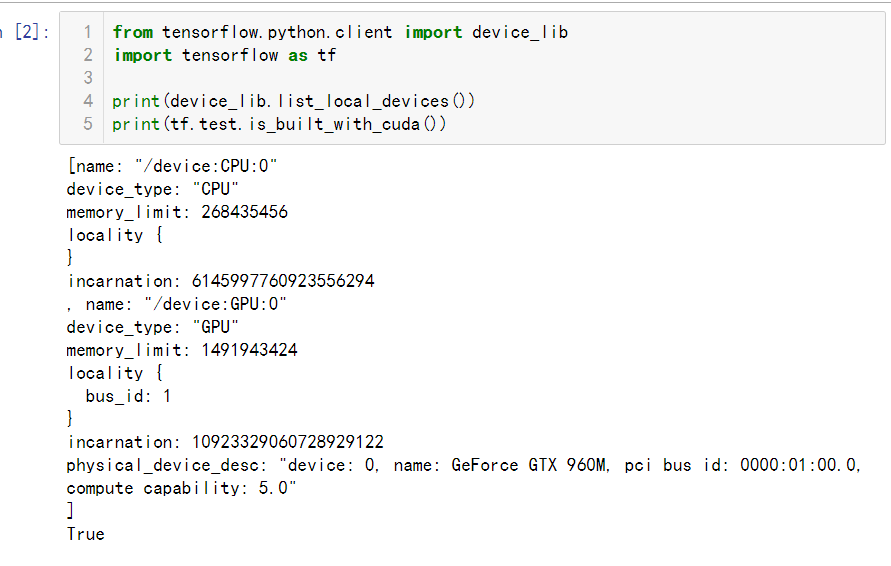# 开始细节步骤：

## (3). cuda安装

https://blog.csdn.net/IT_xiao_bai/article/details/88342921

cuda v9.0安装包下载 ,还有安装指南之类的，稍微看下就行。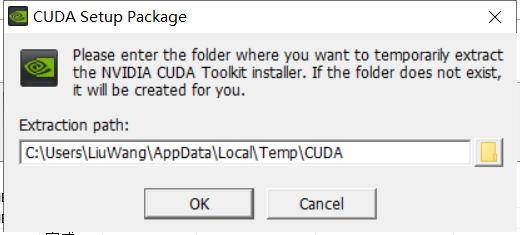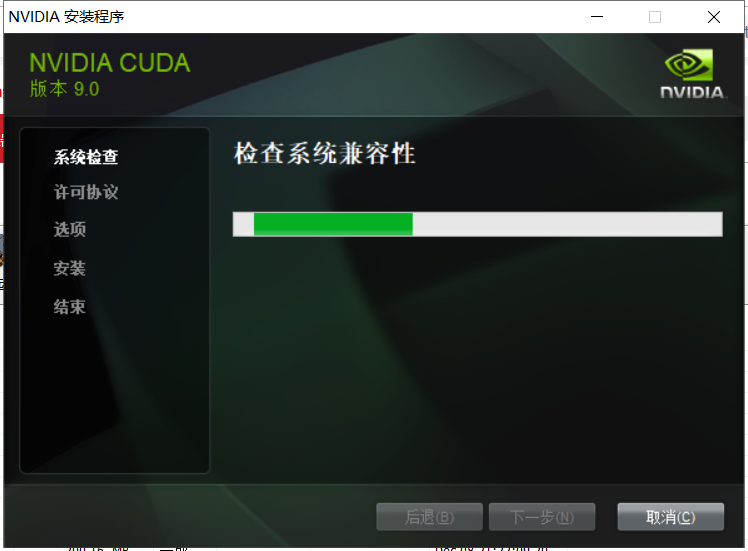NVCC -V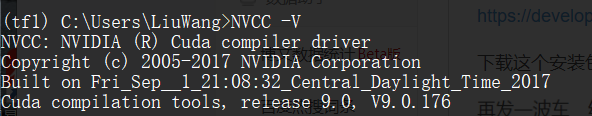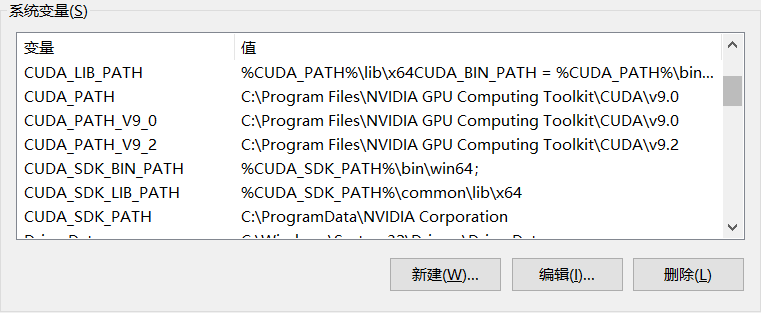(2).cuDnn库下载

https://developer.nvidia.com/cudnn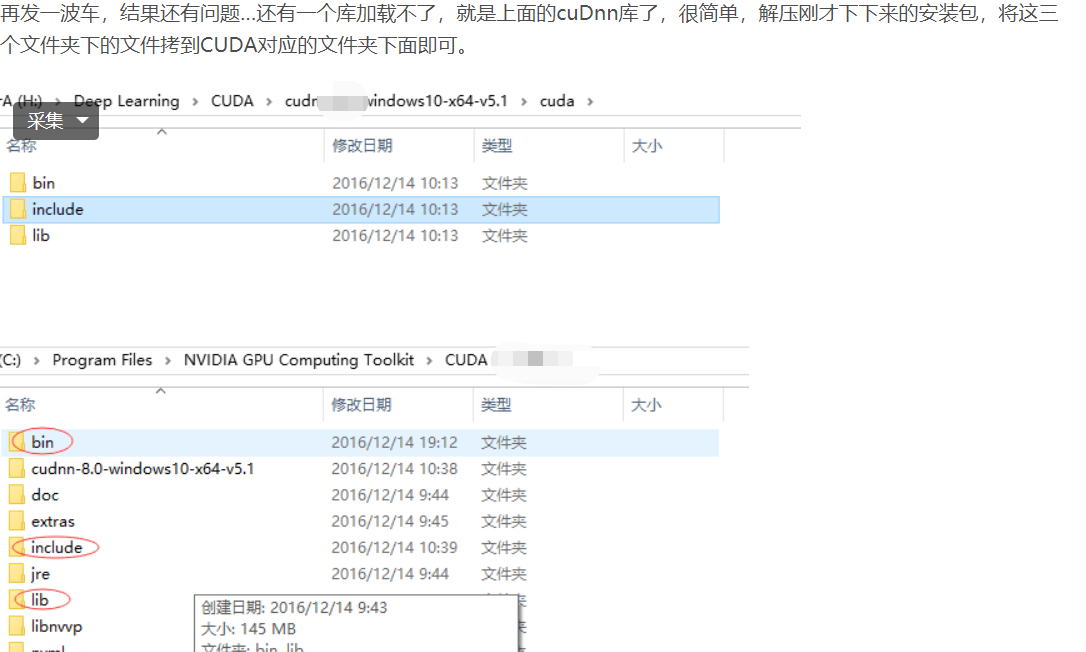(3).cuda测试

(4).关键坑（运行TensorFlow）报错：/envs/tensorflow/lib/python3.5/site-packages/tensorflow/python/framework/dtypes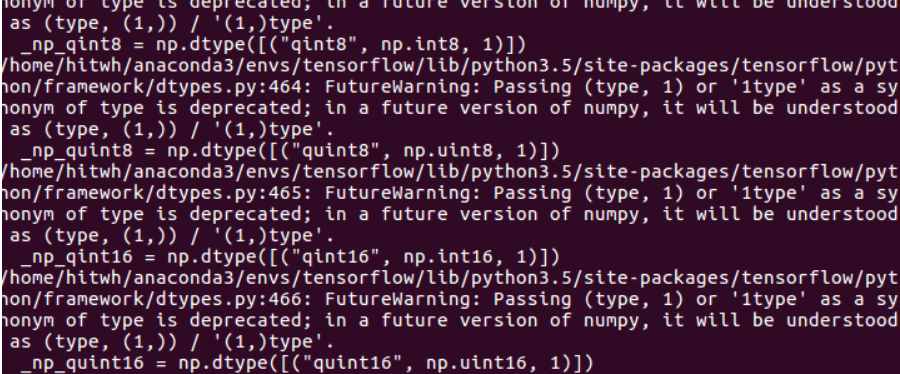``_np_qint8 = np.dtype([("qint8", np.int8,(1,))])_np_quint8 = np.dtype([("quint8", np.uint8,(1,))])_np_qint16 = np.dtype([("qint16", np.int16,(1,))])_np_quint16 = np.dtype([("quint16", np.uint16,(1,))])_np_qint32 = np.dtype([("qint32", np.int32,(1,))])``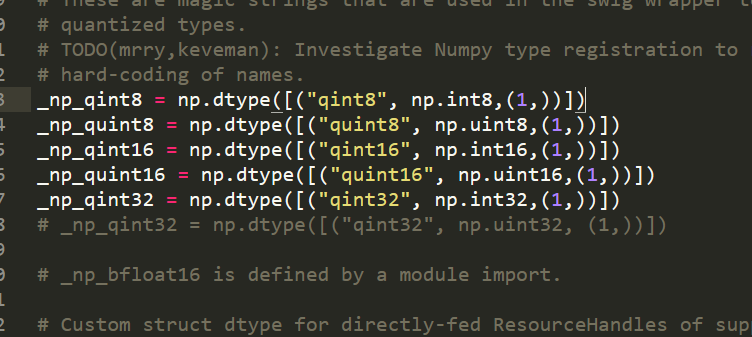``from tensorflow.python.client import device_libimport tensorflow as tf print(device_lib.list_local_devices())print(tf.test.is_built_with_cuda())``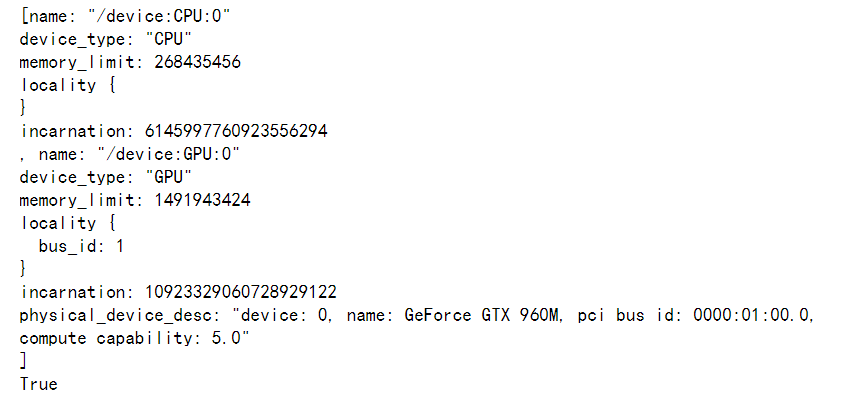https://blog.csdn.net/hsqaihkl/article/details/80674912

https://blog.csdn.net/MahoneSun/article/details/80809042

LInux 版本待跟新 ing...